Hermitian matrices

A Hermitian matrix (or self-adjoint matrix) is a square matrix with complex elements which is equal to its own conjugate transpose — that is, the element in the$i$th row and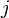$j$th column is equal to the complex conjugate of the element in the$j$th row and$i$th column, for all indices i and j: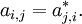$a_{i,j} = a_{j,i}^*.$

If the conjugate transpose of a matrix$A$ is denoted by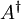$A^\dagger$, then this can concisely be written as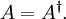$A = A^\dagger. \,$

For example,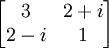$\begin{bmatrix}3&2+i\\ 2-i&1\end{bmatrix}$

All eigenvalues of a Hermitian matrix are real, and, moreover, eigenvectors with distinct eigenvalues are orthogonal. The typical example of a Hermitian matrix in physics is the Hamiltonian (specially in quantum mechanics).